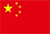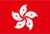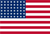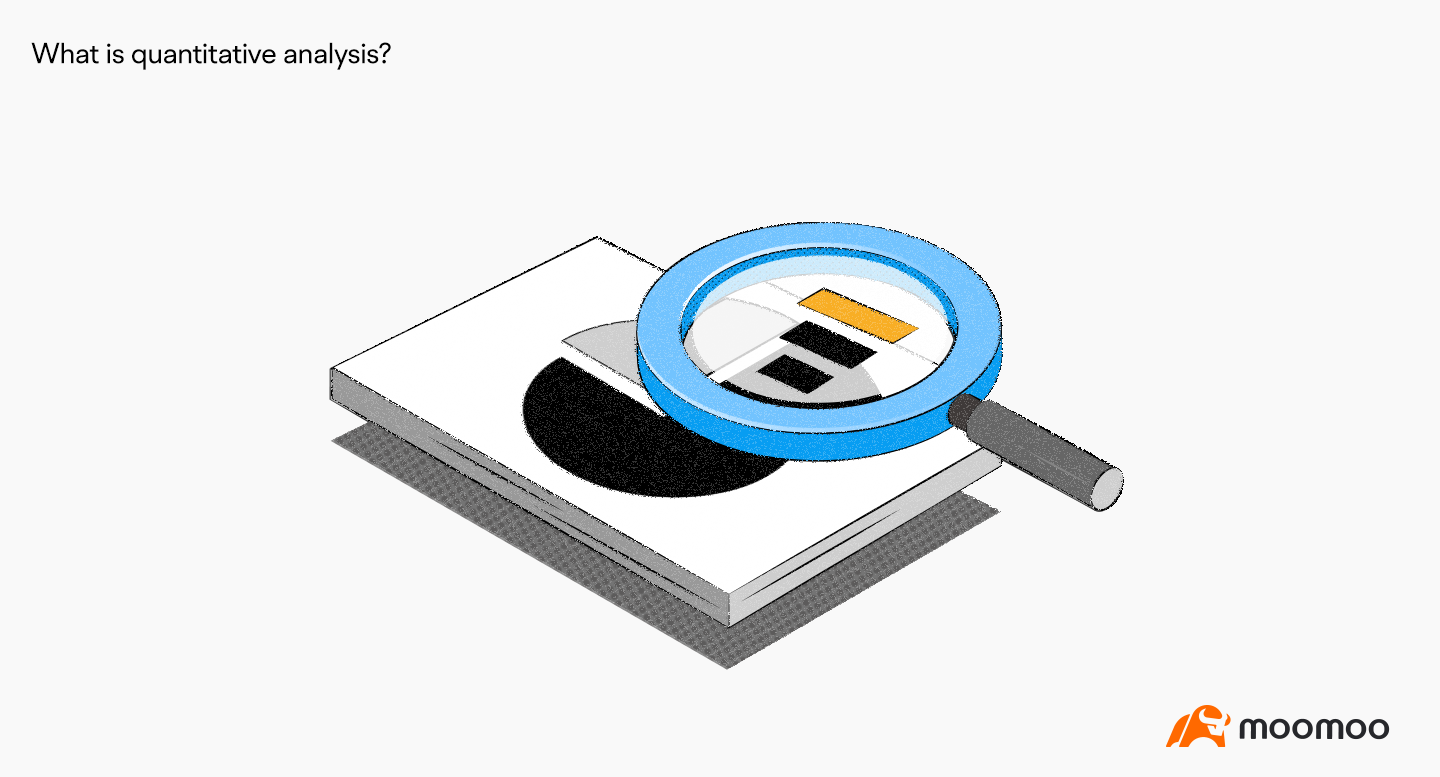Scanning QR Code+
Congratulations
You've successfully got advanced quotes worth 5000 HKD
• Home
• Quotes
• News
• Community
• Learn
• Help Center
• Language
•••EN

# What Is Quantitative Analysis?

Views 84072022.09.28# Key points

● quantitative analysis method is a method to analyze the quantitative characteristics, relations and changes of the research object.

The object of ● quantitative analysis is mainly the financial statements of enterprises.

In ● quantitative analysis, the mathematical model method represents the development direction of quantitative analysis.

# Concept understanding

Quantitative analysis, also known as quantitative analysis, is a method to analyze the quantitative characteristics, quantitative relations and quantitative changes of the research object.

Securities investment analysts often use mathematical models to analyze the quantifiable data of the underlying company. Through quantitative analysis, evaluate the operation of the company and make investment judgment.

The object of quantitative analysis is mainly financial statements, such as balance sheet, profit statement, cash flow statement and so on.

# The basic method of quantitative analysis

Quantitative analysis mainly has the following five basic analysis methods.

1. Ratio analysis. This is also the most important analysis method of quantitative analysis.

2. Trend analysis. Make a vertical comparison of the data of the relevant financial indicators of the same unit for several consecutive years, and observe their growth. Through trend analysis, analysts can understand the development trend of the enterprise in specific aspects.

3. Structural analysis. Through the analysis of the proportion or composition of each sub-project in the enterprise financial indicators in the overall project, consider the status of each sub-project in the overall project.

4. the method of mutual comparison. Through the mutual comparison of economic indicators to reveal the quantitative differences between economic indicators, it can be not only the vertical comparison between the current period and the previous period, but also the horizontal comparison between different enterprises in the same industry, but also can be compared with the standard value. Find out the gap by comparison. and then analyze the reasons for the gap.

5. Mathematical model method. In modern management science, mathematical models are widely used, especially in economic forecasting and management. Because they can not be verified by experiments, mathematical models are usually used to analyze and predict the possible results of economic decisions.

Among the above quantitative analysis methods, the ratio analysis method is the foundation, the trend analysis, structural analysis and comparative analysis are the extension, and the mathematical model method represents the development direction of quantitative analysis.

Disclaimer: The above content does not constitute any act of financial product marketing, investment offer, or financial advice. Before making any investment decision, investors should consider the risk factors related to investment products based on their own circumstances and consult professional investment advisors where necessary.